Study Guide

# Integrating the Velocity Function

## Integrating the Velocity Function

### When Velocity is Non-Negative

Again, let's assume we're cruising on the highway looking for some gas station nourishment. We could actually figure out the distance we've travelled by knowing our velocity and the time we spent searching.

We can only use the formula

distance = velocity × time

if velocity is constant on the time interval we're looking at. If we graph time on the horizontal axis and (constant) velocity on the vertical axis, we get this picture: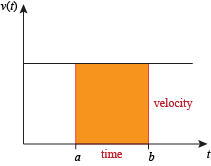The area of this rectangle is velocity × time, which equals distance. The area of this rectangle also happens to be the definite integral of the (constant) velocity function on [a, b]. In symbols, when velocity is constant and positive on [a, b], the distance travelled from t = a to t = b is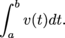### Sample Problem

A car is traveling at v(t) = 60 mph. How far does the car travel in 20 minutes?

20 minutes is one third of an hour, soThis is the area between the graph of the constant function v(t) = 60 and the t-axis on the interval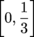(or on any other interval of length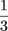hour).

If velocity isn't constant it's not clear how to apply the formula

distance = velocity × time.

What do we use for velocity? One approach is to pretend the velocity is constant: pick one reasonable value for the velocity and pretend that's the velocity for the whole time interval.

If we want to get a better estimate of distance travelled, we can split up the time interval into sub-intervals and pretend that velocity is constant on each sub-interval.

### Sample Problem

Suppose Jen's velocity in mph was measured every ten minutes for one hour, and that her velocity was decreasing over that hour. The recorded values are shown in the table below. Estimate how far Jen travelled during the hour.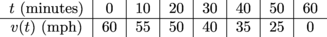We don't know how fast Jen was going at every minute of the hour, but we can pretend that she was going 60 mph for the first ten minutes. Similarly, we can pretend she was going

55 mph from t = 10 to t = 20 minutes

50 mph from t = 20 to t = 30 minutes

40 mph from t = 30 to t = 40 minutes

35 mph from t = 40 to t = 50 minutes

25 mph from t = 50 to t = 60 minutes.

In real life her velocity would probably look something like this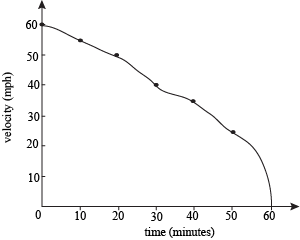but to make the problem easier we're pretending her velocity looks like this: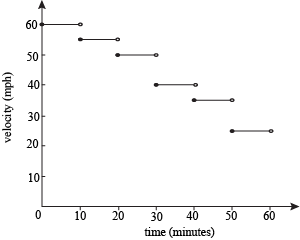Using the formula

distance = velocity × time

on each ten-minute sub-interval, we estimate thatAdding up the estimated distance for each 10-minute sub-interval, we estimate that over the full hour Jen travelled

10 + 9.2 + 8.3 + 6.7 + 5.8 + 4.2 = 44.2 miles.

On each sub-interval we're approximating Jen's velocity. She wasn't going 60 mph for all of the first ten minutes, so this answer is an estimate of how far Jen actually travelled.

In the example above, what we really did was use a left-hand sum with 6 sub-intervals to estimate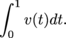The upper limit of integration is 1 because the function v(t) expects t to be in hours.

When we use values of the velocity function and a right- or left-hand sum to approximate distance travelled from time t = a to time t = b, we're also approximating the integral of the velocity function on [a, b].

If the velocity function looks like this: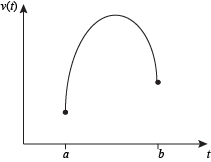and we pretend it looks like this: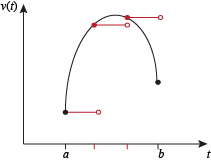then the area of the rectangle on each sub-interval is our estimate of how far the cheetah or snail or whatever has travelled during that sub-interval.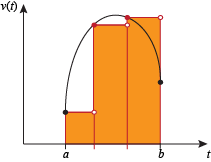As our velocity measurements get closer together, our estimates approach the real distance travelled. Our estimates are also approaching the integral of the velocity function. Since our estimates can't approach two different things, the real distance travelled and the integral of the velocity function must be the same. In symbols, when v(t) is non-negative,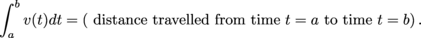• ### Negative Velocity

Velocity is a vector, meaning it has both magnitude and direction. The "direction" of velocity is either positive or negative. Positive and negative velocities describe motion in opposite directions. In Dance Dance Revolution, negative would either be juking left or back and positive would be moving towards the forward and right arrows. However, if the player would rotate 90 degrees, the relative negative and positive directions might change. DDR would enter bazaar-o world, but it could happen. The context of a problem will tell you how to interpret positive and negative velocities for that problem.

### Sample Problems

1) Let v(t) be the velocity of a bug crawling back and forth on the x-axis. When v(t) is positive, the bug is moving to the right:

When v(t) is negative, the bug is moving to the left.

2) Calvin is biking away from his house with velocity v(t).

When v(t) is negative it means Calvin is biking back towards his house.

3) A bird is flying North with velocity v(t).

When v(t) is negative it means the bird is flying South.

Speed is the magnitude, or absolute value, of velocity. This means speed can't be negative!

### Sample Problem

Let v(t), in units per second, be the velocity of a bug crawling back and forth on the x-axis. When v(t) = -5 it means the bug is crawling left at a speed of 5 units per second.

When a question asks "how fast" something is going, you're being asked for the speed.

### Sample Problem

When Calvin's velocity is -7 miles per hour, how fast is he going?

The question is asking for Calvin's speed, which is 7 miles per hour. The negative sign tells which direction Calvin is traveling, but doesn't have anything to do with his speed.

Now that we know what negative velocities mean, it's time to bring the integrals into it again and figure out what integrals of negative velocities mean.

When velocity is non-negative, we know that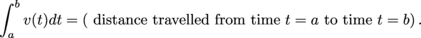When velocity is negative, the integral of velocity is also negative. We can think of this negative value as a weighted distance.

The number part tells the distance, and the negative sign tells the direction, which is the opposite of the direction travelled when v(t) is positive.

Take the area between v(t) and the t-axis that's above the axis. This is the total distance the bug travels to the right.

Take the area that's between v(t) and the t-axis, below the t-axis. This is the total distance the bug travels to the left.

Subtract:

(distance bug travels right) – (distance bug travels left) = 9 – 19 = -10.

This is the net change in the bug's position from time t = 0 to time t = 10.

We found this net change by taking a weighted sum of the areas between v(t) and the t-axis, which means we also just found the integral of v(t) from 0 to 10: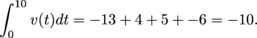Whatever the units, when velocity is 0, speed is also 0. If something isn't moving at all, it's not moving in any direction. If v(t) is Calvin's velocity away from his house, measured in mph, then when v(t) = 0 Calvin isn't moving away from his house and he's not moving towards his house either.

• ### Change in Position

Let v(t) be a velocity function on the time interval [a, b]. The function v(t) describes the movement of something&mdash;maybe a car, maybe an emu, maybe a banana slug. The banana slug is at some starting position when t = a, travels some distance from t = a to t = b, and is at some ending position when t = b.

If v(t) ≥ 0 on [a, b], then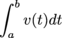is positive and is the distance travelled between time t = a and time t = b.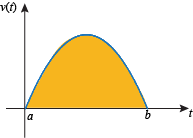We could also say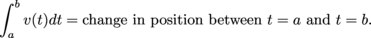If v(t) ≤ 0 on [a, b], then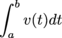is negative and is the distance travelled between time t = a and t = b, but in the "opposite" direction.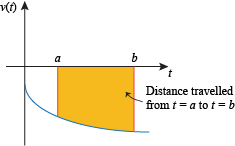In other words,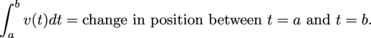If v(t) is sometimes negative and sometimes positive on [a, b], thenis the distance travelled in the positive direction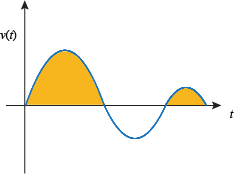minus the distance travelled in the negative direction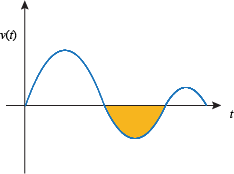We can also describe this by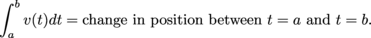Regardless of whether the velocity function is positive, negative, or a little bit of each,We're going to introduce a new function s(t) so that we can write this equation more compactly. Let

s(t) = position at time t.

So s(a) = position at time t = a, s(b) = position at time t = b, etc.

On the interval [a, b], s(a) is the position of the car or cheetah at the start of the interval and s(b) is the position of the car or cheetah at the end of the interval. The change in position of the car or cheetah over the interval [a, b] is

(ending position) – (starting position) = s(b) – s(a)

However, the change in position of the car or cheetah over the interval [a, b] is also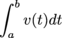. This means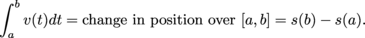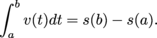We'd like to point out that velocity is the derivative of position (change in position with respect to time). In symbols,

v(t) = s'(t).

For the following examples and exercises, assume s(t) is the position function and v(t) = s'(t) is the velocity function.

The equation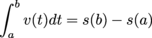describes a relationship between the three values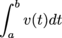, s(a), and s(b). Most problems involving this equation will give you two of these values and ask you to find the third.

You could be given the value of the integral and one of the values s(a) or s(b).

We've been working with the equationin this form because this is how the Fundamental Theorem of Calculus is usually given. However, we can also rearrange the equation

by adding s(a) to both sides to get this: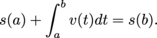This rearranged equation says that if you take the starting position (s(a)) and add the change in position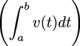you get the ending position, s(b).

Some problems are easier if we use the rearranged equation, especially those where we're given s(a) and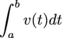and asked to find s(b).

• ### Total Distance Travelled vs. Change in Position

There's a difference between how far someone travels, and the change between their starting and ending positions. Take a basketball player running suicide drills. He travels to one line and back to his starting point, then to a farther line and back to his starting point, and does that several times. By the time he's done, he's certainly travelled some distance. However, since he ends up back where he started, his change in position is 0.

When we integrate a velocity function from t = a to t = b, the number we get is the change in position between t = a and t = b. If we want to know the total distance travelled, we have to do something different. We don't want travel in opposite directions canceling out, so we find how far we travel in one directionand how far we travel in the opposite directionand then, instead of subtracting, we add these distances together. We're pretending all the distances travelled are in the same direction. Another way to think of this is that we're integrating the absolute value of the velocity function, |v(t)|.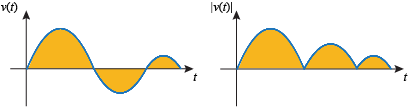This gets us the total (unweighted) area between the function v(t) and the horizontal axis.

### Sample Problem

A cat climbs a tree with velocity given by the graph below.If the cat starts at ground level (height s(0) = 0 feet),

(a) how high is the cat when t = 8 seconds?

(b) what is the total distance the cat travels from t = 0 to t = 8?

(a) This part of the question is like ones we did earlier. We want to know the cat's change in position from t = 0 to t = 8, so we integrate the velocity function by looking at the areas on the graph. We find that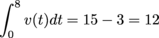so the cat's position at t = 8 is s(8) = 12 feet.

(b) This part of the question is asking for the total distance the cat traveled, which means we want to find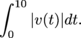.

To do this we count all the areas between the graph of v(t) and the horizontal axis positively, to get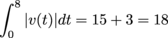This means the cat traveled a total distance of 18 feet.